# The Mathematical Experience

### by Philip J Davis & Reuben Hersh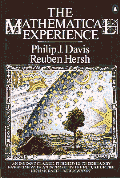#### 4.Proof (p147)

The assertion has been made that mathematics is uniquely characterised by something known as "proof".The first proof in the history of mathematics is said to have been given by Thales of Miletus (600BC).He proved that the diameter divides circles into two equal parts.Now this is a statement which is so simple that it appears self evident.The genius of the act was to understand that a proof was possible and necessary.What makes a mathematical proof more than mere pedantry is its application in situations where the statements made are far less transparent.In the opinion of some,the name of the mathematics game is proof;no proof,no mathematics.In the opinion of others,this is nonsense;there are many games in mathematics.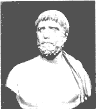Thales c.624-548 B.C.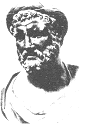Pythagoras c.580-500 B.C.

To discuss what proof is,how it operates,and what it is for,we need to have a concrete example of some complexity before us;and there is nothing better than to take a look at what undoubtedly is the most famous theorem in the history of mathematics.We allude to the Pythagorean Theorem,as it occurs in Proposition 47.Book 1 of Euclid's Elements (300BC).We quote it in the English version given by Sir Thomas Heath.The in-text numbers at the right are references to previously established results or to "common notions."

Proposition 47 In the right-angled triangles the square on the side subtending the right angle is equal to the squares on the sides containing the right angle.

Let ABC be a right-angled triangle having the angle BAC right;

I say that the square on BC is equal to the squares on BA,AC.

For let there be described on BC,the square BDEC,and on BA,AC the squares GB,HC;[1.46] through A let AL be drawn parallel to either BD or CE,and let AD,FC be joined.

Then,since each of the angles BAC,BAG is right,it follows that with a straight line BA,and at the point A on it,the two straight lines AC,AG not lying on the same side make adjacent angles equal to two right angles;therefore CA is in a straight line with AG.[1.14]

For the same reason BA is also on a straight line with AH.And,since the angle DBC is equal to the angle FBA;for each is right:let the angle ABC be added to each;therefore the whole angle DBA is equal to the whole angle FBC.[C.N.2]

And,since DB is equal to BC,and FB to BA,the two sides AB,BD are equal to the two sides FB,BC respectively,and the angle ABD is equal to the angle FBC:therefore the base AD is equal to the base FC,and the triangle ABD is equal to the triangle FBC.[1.4]

Now the parallelogram BL is double of the triangle ABD,for they have the same base BD and are in the same parallels BD,AL. [1.41]

And the square GB is double of the triangle FBC,for they again have the same base FB are are in the same parallels FB,GC.[1.41]

[But the doubles of equals are equal to one another.]

Therefore the parallelogram BL is also equal to the square GB.

Similarly,if ARE,BK be joined,the parallelogram CL can also be proved to be equal to the square HC;therefore the whole square BDEC is equal to the two squares GB,HC.[C.N.2]

And the square BDEC is described on BC,and the squares GB,HC on BA,AC.

Therefore the square on the side BC is equal to the squares on the sides BA,AC.

Therefore etc. Q.E.D.

Now,assuming that we have read Euclid up to Proposition 47,and assuming we are intellectually able to get through his material,what are we to make of it all? Perhaps the most beautiful recorded reaction is that ascribed to Thomas Hobbes (1588-1679) [Ref : Analysis10; Langton {Mimic.wav}] by John Aubrey in his "Brief Lives":

He was 40 yeares old before he looked on Geometry;which happened accidentally.Being in a Gentleman's Library, Euclid's Elements lay open,and 'twas the 47 El.libri 1.He read the Proposition.By G__,sayd he (he would now and then sweare and emphatical Oath by way of emphasis) this is impossible! So he reads the Demonstration of it,which referred him back to such a Proposition;which proposition he read.That referred him back to another,which he also read.Et sic dienceps [and so on] that at last he was demonstrably convinced of that trueth. That made him in love with Geometry.

What appears initially as unintuitive,dubious,and somewhat mysterious ends up,after a certain kind of mental process,as gloriously true.Euclid,one likes to think,would have been proud of Hobbes and would use him as Exhibit A for the vindication of the proof process,discovered and promulgated by Greek mathematics,in the service of validation and certification.Now that the statement has been proved,we are to understand that the statement is true beyond the shadow of a doubt.

The backward referral to previous propositions,alluded to by Hobbes,is characteristic of the method of proof,and as we know,this can't go on forever.It stops with the so-called axioms and definitions.Whereas the latter are mere linguistic conventions,the former represent rock bottom self-evident facts upon which the whole structure is to rest,held together by the bolts of logic.

Also characteristic of the method is the considerable degree of abstraction that has occurred in the refinement of such concepts as triangle,right angle,square,etc.The figure itself appears here as a very necessary adjunct to the verbalisation.In Euclid's presentation we cannot wholly follow the argumentation without the figure in our mind's eye,we would be reduced to supplying our own figure if the author had not done it for us.Notice also that the language of the proof has a formal and severely restricted quality about it.This is not the language of history,nor of drama,nor of day to day life,this is language that has been sharpened and refined so as to serve the precise needs of a precise but limited intellectual goal.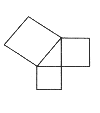One response to this material was recorded by the poet Edna Millay,in her line,"Euclid alone has looked on Beauty bare." A shudder might even run down our spines if we believe that with a few magic lines of proof we have compelled all the right triangles in the universe to behave in a regular Pythagorean fashion.

Abstraction,formalization,axiomatization,deduction - here are the ingredients of proof.And the proofs in modern mathematics,though they deal with different raw material or lie at a deeper level,have essentially the same feel to the student or the researcher as the one just quoted.

Further reading of Euclid's masterpiece brings up additional issues.Notice that in the figure certain lines eg BK,AL,seem to be extraneous to a minimal figure drawn as an expression of the theorem itself.Such a figure is illustrated here:a right angled triangle with squares drawn upon each of its three sides.The extraneous lines which in high school are often called "construction lines," complicate the figure,but form an essential part of the deductive process. They reorganise the figure into subfigures and the reasoning takes place precisely at this sublevel.

Now,how does one know where to draw these lines so as to reason with them? It would seem that these lines are accidental or fortuitous.In a sense this is true and constitutes the genius or the trick of the thing.Finding the lines is part of finding the proof,and this may be no easy matter.With experience come insight and skill at finding proper construction lines.One person may be more skilful at it than another.There is no guaranteed way to arrive at a proof.This sad truth is equally rankling to schoolchildren and to skilful professionals.Mathematics as a whole may be regarded as a systematisation of just those questions which have been pursued successfully.

Mathematics,then,is the subject in which there are proofs.Traditionally,proof was first met in Euclid;and millions of hours have been spent in class after class,in country after country,in generation after generation,proving and reproving the theorems of Euclid.After the introduction of the "new math" in the mid-nineteen fifties,proof spread to other high school mathematics such as algebra,and subjects such as set theory were deliberately introduced so as to be a vehicle for the axiomatic method and proof.In college,a typical lecture in advanced mathematics,especially a lecture given by an instructor with "pure" interests,consists entirely of definition, theorem, proof, definition, theorem,proof,in solemn and unrelieved concatenation. Why is this? If,as claimed,proof is validation and certification,then one might think that once a proof has been accepted by a competent group of scholars,the rest of the scholarly world would be glad to take their word for it and to go on.Why do mathematicians and their students find it worthwhile to prove again and yet again the Pythagorean Theorem or the theorems of Lebesgue or Weiner or Kolmogoroff?

Proof serves many purposes simultaneously.In being exposed to the scrutiny and judgment of a new audience,the proof is subject to a constant process of criticism and revalidation. Errors,ambiguities,and misunderstandings are cleared up by constant exposure.Proof is respectability.Proof is the seal of authority.

Proof,in its best instances,increases understandings by revealing the heart of the matter. Proof suggests new mathematics.The novice who studies proof gets closer to the creation of new mathematics.Proof is mathematical power,the electric voltage of the subject which vitalises the static assertions of the theorems.

Finally,proof is ritual,and a celebration of the power of pure reason.Such an exercise in reassurance may be very necessary in view of all the messes that clear thinking clearly gets us into.

R.Blanché:J.Dieudonné ;G.H.Hardy;T.Heath;R.Wilder 

#### 4.Infinity,or the Miraculous Jar of Mathematics (p152)

Mathematics,in one view,is the science of infinity.Whereas the sentences "2+3 = 5", "1/2+1/3 = 5/6", "seventy-one is a prime number", are instances of finite mathematics, significant mathematics is thought to emerge when the universe of its discourse is enlarged so as to embrace the infinite. The contemporary stockpile of mathematical objects is full of infinities.The infinite is hard to avoid.Consider a few typical sentences: "there are an infinite number of points on the real line,"lim n®¥ n/n+1=1," å¥n=11/n2 = p2/6," "S¥0 sinx/x dx = p/2," "À0 + 1 = À0," "The number of primes is infinite," "is the number of twin primes infinite?," "the tape on a Turing machine is considered to be of infinite extent," "let N be an infinite integer extracted from the set of hyperreals." We have infinities and infinities upon infinities; infinities galore, infinities beyond the dreams of conceptual avarice.
The simplest of all the infinite objects is the system of positive integers 1,2,3,4,5,..... The "dot dot dot" here indicates that the list goes on forever. It never stops. This system is commonplace and so useful that it is convenient to give it a name. Some authors call it N (for the numbers) or Z (for Zahlen,if they prefer a continental flavour).The set N has the property that if a number is in it, so is its successor. Thus,there can be no greatest number,for we can always ad one and get a still greater one. Another property that N has is that you can never exhaust N by excluding its members one at a time. If you delete 6 and 83 from N,what remains is an infinite set. The set N is an inexhaustible jar, a miraculous jar recalling the miracle of the loaves and the fishes in Matthew 15:34.
This miraculous jar with all its magical properties,properties which appear to go against all the experiences of our finite lives,is an absolutely basic object in mathematics,and thought to be well within the grasp of children in the elementary schools.Mathematics asks us to believe in this miraculous jar and we shan't get far if we don't.
It is fascinating to speculate on how the notion of the infinite breaks into mathematics. What are its origins? The perception of great stretches of time? The perception of great distances such as the vast deserts of Mesopotamia or the straight line to the stars? Or could it be the striving towards ultimate but realizable explanations?
The infinite is that which is without end. It is the eternal,the immortal,the self-renewable,the apeiron of the Greeks,the ein-sof of the Kaballah, the cosmic eye of the mystics which observes us and energizes us from the godhead.
Observe the equation

1/2 + 1/4 +1/8 +1/16 +................=1,

or,in fancier notation å¥n=1 2-n =1.On the left-hand side we seem to have incompleteness,infinite striving. On the right-hand side we have finitude,completion. There is a tension between the two sides which is a source of power and paradox. There is an overwhelming mathematical desire to bridge the gap between the finite and the infinite. We want to complete the incomplete,to catch it,to cage it,to tame it.*

[* Kumen describes a game in which two mathematicians with a deep knowledge of the infinite try to outbrag each other in naming a greater cardinal number than their opponent. Of course,one can always add one to get a yet higher number,but the object of the game as played by these experts is to score by breaking through with an altogether new paradigm of cardinal formation. The playoff goes something along these lines: XVII, 1,295,387, 101010,w, W(ww), The first inaccessible cardinal, The first hyper-inaccessible cardinal, The first Mahlo cardinal, The first hyper-Mahlo,The first weakly compact cardinal,The first ineffable cardinal.
Obviously it would not be cricket to name a number that doesn't exist,and a central problem in large cardinal theory is precisely to explicate the sense in which the above mentioned hypers and ineffables exist.]

Mathematics thinks it has succeeded in doing this. The unnamable is named [As that is the mother of all things-LB] ,operated on, tamed, exploited, finitized, and ultimately,trivialized [Which is why people don't like it-LB]. Is,then, the mathematical infinite a fraud? Does it represent something that is not really infinite at all? Mathematics is expressible in language that employs a finite number of symbols strung together in sentences of finite length. Some of these sentences seem to express facts about the infinite. Is this a mere trick of language wherein we simply identify certain types of sentences  as speaking about "infinite things"? when infinity has been tamed it enjoys a symbolic life.
Cantor introduced the symbol À0  ("aleph nought") for the infinite cardinal number represented by the set of natural numbers, N. He showed that this number obeyed laws of arithmetic quite different from those of finite numbers;for instance À0 + 1 = À0, À0 + À0 = À0, etc.
Now,one could easily manufacture a hand-held computer with a À0 button to obey the Cantorian laws. But if  À0 has been encased algorithmically with a finite structure, in what,then,consists its infinity? Are we dealing only with so-called infinities? We think big and we act small. We think infinities and we compute finitudes. This reduction, after the act,is clear enough,but the metaphysics of the act is far from clear.
Mathematics,then,asks us to believe in ana infinite set. What does it mean that an infinite set exists? Why should one believe in it? In formal presentation this request is institutionalized by axiomitization. Thus,in Introduction to Set Theory,by Hrbacek and Jceh,we read on page 54:
"Axiom of Infinity. An inductive (ie infinite) set exists."
Compare this against the axiom of God as presented by Maimonides (Misneh Torah,Book 1,Chapter 1):

The basic principle of all basic principles and the pillar of all the sciences is to realize that there is a First Being who brought every existing thing into being.

Mathematical axioms have the reputation of being self-evident,but it might seem that the axioms of infinity and that of God have the same character as far as self-evidence is concerned. which is mathematics and which is theology? Does this,then,lead us to the idea that an axiom is merely a dialectical position on which to base further argumentation,the opening move of a game without which the game cannot get started?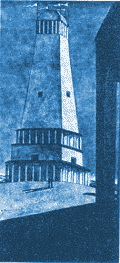Exposure to the ideas of modern mathematics has led artists to attempt to depict graphically the haunting qualities of the infinite. De Chirico.Nostalgia of the Infinite. 1913-14? (dated 1911 on the painting) Courtesy:Museum of Modern Art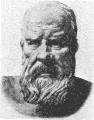Galileo Galilei 1564-1642

The paradox of Achilles and the tortoise asserts that Achilles cannot catch up with the tortoise,for he must first arrive at the point where the tortoise has just left,and therefore the tortoise is always in the lead.
Galileo's paradox says that there as as many square numbers as there are integers and vice versa. This is exhibited in the correspondence

 1 2 3 4 5........ 1 4 9 16 25......

Yet,how can this be when not every number is a square?
The paradoxes of rearrangements say that the sum of an infinite series may be changed by rearranging its terms.
For example,

0 = (1-1) + (1-1) + (1-1) + (1-1) + ........ = 1 + (-1+1) + (-1+1) +....= 1 + 0 + 0 +.........=1.

Dirac's function d(x) carries the definition

d(x) = 0 if x ¹ 0, d(0) = ¥, S ¥ d(x) dx = 1,

which is self-contradictory within the framework of classical analysis.
Achilles is an instance of irrelevant parameterization: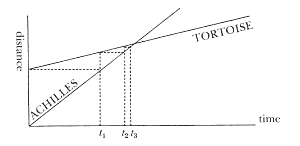Of course,the tortoise is always ahead at the infinite sequence of time instants t1,t2,t3...where Achilles has just managed to catch up to where the tortoise was at the last time instant.So what? Why limit our discussion to the convergent sequence of times t1,t2.....? This is a case of the necessity of keeping one's eye on the doughnut and not on the hole.

Galileo's paradox is regularized by observing that the phenomenon it describes is a distinguishing characteristic of an infinite set. An infinite set is, simply, a set which can be put into one to one correspondence with a proper subset of itself. Infinite arithmetic is simply not the same as finite arithmetic. If Cantor tells us that À0 + 1 = À0 is a true equation, one has no authorization to treat À0 as a finite quantity, subtract it from both sides of the equation to arrive at the paradoxical statement 1 = 0.
Berkeley's paradox of the infinitesimals was first ignored,then bypassed by phrasing all calculus in terms of limiting processes. In the last decade it has been regularized by "nonstandard" analysis in a way which seems to preserve the original flavour of the creators calculus.
The paradoxes of rearrangement, aggregation, noncompactness are now dealt with on a day to day basis by qualification and restrictions to absolutely convergent series, absolutely convergent integrals,uniform convergence, compact sets. The wary mathematician is hedged in, like a slalom skier, by hundreds of flags within whose limits he must run his course.
The Dirac paradox which postulates the existence of a function with contradictory properties is squared away by the creation of a variety of operational caculii such as that of Temple-Lighthill or Mikusinski or, the most notable, Schwartz's theory of distributions (generalized functions).
By a variety of means,then the infinite has been harnessed and then housebroken. But the nature of the infinite is that it is open-ended and the necessity for further cosmetic acts will always reappear.

Further Readings. See Bibliography
B. Bolzano; D. Hilbert; K Kuhnen ; L.Zippin.

#### 4.The Stretched String (p158)

In the standard formalization of mathematics, geometry is reduced, by the use of coordinates, to algebra and analysis. These in turn, by familiar constructions of set theory, are reduced to numbers. This is done because it came to be thought by the end of the nineteenth century that the intuitive notion of the natural numbers was crystal-clear, whereas the notion of a continuous straight-line segment seems more subtle and obscure the more we study it. Nevertheless, it seems clear that historically and psychologically the intuitions of geometry are more primitive than those of arithmetic.

In some primitive cultures there are no number words except one, two, and many. But in every human culture that we will ever discover, it is important to go from one place to another, to fetch water or dig roots. Thus human beings were forced to discover-not once, but over and over again, in each new human life-the concept of the straight line, the shortest path from here to there, the activity of going directly towards something.

In raw nature, untouched by human activity , one sees straight lines in primitive form. The blades of grass or stalks of corn stand erect, the rock falls down straight, objects along a common line of sight are located rectilinearly.

But nearly all the straight lines we see around us are human artifacts put there by human labor. The ceiling meets the wall in a straight line, the doors and window-panes and tabletops are all bounded by straight lines. Out the window one sees rooftops whose gables and corners meet in straight lines, whose shingles are layered in rows and rows, all straight.

The world, so it would seem, has compelled us to create the straight line so as to optimize our activity, not only by the problem of getting from here to there as quickly and easily as possible, but by other problems as well. For example, when one goes to build a house of adobe blocks, one finds quickly enough that if they are to fit together nicely,their sides must be straight. Thus the idea of a straight line is intuitively rooted in the kinesthetic and the visual imaginations. We feel in our muscles what it is to go straight toward our goal, we can see with our eyes whether someone else is going straight.The interplay of these two sense intuitions gives the notion of straight line a solidity that enables us to handle it mentally as if it were a real physical object that we handle by hand.

By the time a child has grown up to become a philosopher, the concept of a straight line has become so intrinsic and fundamental a part of his thinking that he may imagine it as an Eternal Form, part of the Heavenly Host of Ideals which he recalls from before birth. Or, if his name be not Plato but Aristotle, he imagines that the straight line is an aspect of Nature, an abstraction of a common quality he has observed in the world of physical objects. He tends to forget the extent to which the straight lines that are observed are there because we have invented and built them.

In the course of human physical activity, the straight line enters the human mind, and becomes there a shared concept, the straight line about which we can reason, the mathematical straight line. As mathematicians, we discover that there are theorems to be proved having to do with geodesics-the shortest paths, the solutions of the problem of minimizing the distance from here to there-and having to do with paths of constant curvature - paths which are "unchanged" by sliding them back and forth along themselves.

Just what constitutes the "straightness" of the straight line? There is undoubtedly more in this notion than we know and more than we can state in words or formulas.

Here is an instance of this "more." Suppose a, b, c, d are four points on a line. Suppose b is between a and c, and c is between b and d. Then what can we conclude about a, b, and d ? It will not take you long to conclude that b must lie between a and d.This fact, surprisingly, cannot be proved from Euclid's axioms ; it has to be added as an additional axiom in geometry. This omission of Euclid was first noticed 2000 years after Euclid, by M. Pasch in 1882! Moreover, there are important theorems in Euclid whose complete proof requires Pasch's axiom; without it, the proofs are not valid.

(*H.Guggenheimer has shown that another version of Pasche's axiom can be derived as a theorem using Euclid's Fifth Postulate [Ref: I.Asimov "The Left Hand of the Electron -Ch10"])

This example shows that an intuitive notion may not be completely described by the axioms which are stated in a theory.

The same thing happens in modern mathematics. It was discovered by the Norwegian logician Thorolf Skolem that there are mathematical structures which satisfy the axioms of arithmetic, but which are much larger and more complicated than the system of natural numbers. These "nonstandard arithmetics" may include infinitely large integers. In reasoning about the natural numbers, we rely on our complete mental picture of these numbers. Skolem's example shows that there is more information in that picture than is contained in the usual axioms of arithmetic.

The conclusion that b is between a and d in Pasch' s theorem may seem to be a trivial one. For we get this answer simply by making a little picture with pencil and paper. We follow the directions on how to arrange the dots, and we see that in the end b is between a and d. In other words, we use a line on paper to determine the properties of the ideal line, the mathematical line. What could be simpler? But there are two points that are worth bringing up front, out of the background where they usually lurk. First of all, we know that the ideal line is different from the line on paper.

Some of the properties of the line on paper are "accidental" not shared by the mathematical line. How do we know which ones?

In the figure for Pasch's axiom,we put a,b,c,d somewhere, and get our picture. We could have drawn the figure in many other ways, for we have complete freedom as to how far apart any two points should be. Yet we are sure that the answer will always be the same , b is between a and d. We draw only one picture, but we believe that it is representative of all possible pictures. How do we know that?

The answer has to do with our actually sharing a definite intuitive notion, about which we have some reliable knowledge. However, our knowledge of this intuitive notion is by no means complete-neither in the sense of explicit knowledge, nor even in the sense of providing a basis from which to derive complete information, possibly by very long, difficult arguments.

One of the oldest questions about the straight line (as old as Zeno) is this: Can a segment of finite length be divided into infinitely many parts? To the mathematically-trained reader, the answer is an unhesitating "Yes." It may be suspected that few persons, if any, could be found today who would dispute this point of gospel. But 200 years ago, it was a different story.

Listen to the opinion of George Berkeley on this subject:

. . . . to say a finite quantity or extension consists of parts infinite in number is so manifest a contradiction, that everyone at first sight acknowledges it to be so ; and it is impossible it should ever gain the assent of any reasonable creature who is not brought to it by gentle and slow degrees, as a converted Gentile to the belief of transubstantiation.

If we accept Berkeley as a competent witness as to the eighteenth-century intuitive notion of the straight line,perhaps we should conclude that the question of infinite divisibility was undecidable. By the twentieth century, the weight of two hundred years of successful practice of analysis has settled the question. "Between two distinct points on the line there exists a third point" is intuitively obvious today.

Today the continuum hypothesis (see Chapter 5, Non-Cantorian Set Theory) is an undecidable question about the straight line. This is true in two senses. As a mathematical theorem, the combined work of Gödel and Cohen, it is a statement about the Zermelo-Frankel-Skolem axiom system. It has been proved that neither the continuum hypothesis nor its negation can be proved from these axioms.

The continuum hypothesis is also undecidable in a larger sense - that no one has been able to find intuitively compelling arguments to accept or reject it. Set theorists have been searching for over ten years for some appealing or plausible axiom that would decide the truth of the continuum hypothesis and have not yet found one.

It may be that our intuition of the straight line is permanently incomplete with respect to set-theoretical questions involving infinite sets. In that case, one can add as an axiom either the continuum hypothesis or its negation. We would have many different versions of the straight line, none of which was singled out as intuitively right.

The question of divisibility, it seems, was settled by the historical development of mathematics. Perhaps the continuum hypothesis will be settled in the same way. Mathematical concepts evolve, develop, and are incompletely determined at any particular historical epoch. This does not contradict the fact that they also have well-determined properties, both known and unknown, which entitle them to be regarded as definite objects.

Further Readings. See Bibliography
H. Guggenheimer.

#### 4.The Coin of Tyche (p163)

[Please note,this isn't the coin of Tai-Chi!-LB]
How many really basic mathematical objects are there? One of them is surely the "miraculous jar" of the positive integers 1, 2, 3 Another is the concept of a fair coin. Though gambling was rife in the ancient world and although prominent Greeks and Romans sacrificed to Tyche, the goddess of luck, her coin did not arrive on the mathematical scene until the Renaissance. Perhaps one of the things that had delayed this was a metaphysical position which held that God speaks to humans through the action of chance. Thus, in the Book of Samuel, one learns how the Israelites around 1000 B.C. selected a king by casting lots:

Samuel then made all the tribes of Israel come forward, and the lot fell to the tribe of Benjamin. He then made the tribe of Benjamin come forward clan by clan and the lot fell to the clan of Matri. He then made the clan of Matri come forward man by man and the lot fell to Saul the son of Kish.
1 Samuel 10:20-21

The implication here is that the casting of lots is not merely a convenient way of arriving at a leader, but it is done with divine approbation and the result is an expression of the divine will. Tossing a coin is still not an outmoded device for decision making in the face of uncertainty.

The modern theory begins with the expulsion of Tyche from the Pantheon. There emerges the vision of the fair coin, the unbiased coin. This coin exists in some mental universe and all modern writers on probability theory have access to it. They toss it regularly and they speculate about what they "observe." what do they report? Well, the fair coin is a two-sided affair in splendid mint condition. For convenience, one side is designated as heads (H) and the other as tails (T). The fair coin is tossed over and over again and one observes the outcome. There is also the notion of a fair tosser, i.e., an individual who takes a fair coin and without subterfuge, sleight of hand, or other shady physical manipulation tosses the fair coin. But, for simplicity, let us agree to roll the tossed and the tosser into one abstract concept.

 HHHTHTHHTTTTTTHHHTHTHHTTTHTHTTTH HHTHTHTHTHTTTTHTTTTTTTHHHTTHHTTT TTTHTTTHTHTTHHTHHHHTTHTTTHHTTHHT HTTTHTTHTTTTTTTTTTTTHHTTTTHTTHTT THHHHTHTTHTTTHTTHHHHHTTTHHTHHHHH HHTTTHHTHHHTHTHHHTHHHHTTTHTTHTHH HHHTHTHHTTTTTTHHHTHTHHTTTHTHTTTH HHTHTHTHTHTTTTHTTTTTTTHHHTTHHTTT TTTHTTTHTHTTHHTHHHHTTHTTTHHTTHHT HTTTHTTHTTTTTTTTTTTTHHTTTTHTTHTT THHHHTHTTHTTTHTTHHHHHTTTHHTHHHHH HHTTTHHTHHHTHTHHHTHHHHTTTHTTHTHH HHTTHTHHHTHTTTHTTTTHHHTHHHHTHHTH THHHTHTHHHHHHTHTTTTHTTHTTHHHHHHH TTTTHTHHHTTHHHHHHHHHTHTTHHTHTTTT TTTTTTTTHTTTTHTTHTHHHHHHHHTHTHTH HHHHHTHHTHTHHHTTHTHHTTTHTHHTHTTH THTTHHHTHHHTTTTTHHHHHHTTTTHTTTHT HTHHHTTHHTTHHHTHHTTHTHHTTTTHHHHTH HTHHHHHTHTHHHTTTHHHHTTTTHHHTHTTTH THTHTHTHHTHHTTHTTTTHTHTHHHHHHHHHH TTTTHHHTTTTHHTHHTTHTTTTTHHTTTHHHHH TTTHTHHHTHTTTHHTTHHTHTTHHHHHHTHTHT TTTHHTTTHTTTHTHTTTHTTTHHTTTHHHTH HTTHHHHHTTHHHHTTHHTTTTTHHHTTTTHT HTHTTHTHTHHHTHTTTTHHHHHHTTTTHTHT HHTHHHTTHHHHHHHTHTTHHHHHHTTTTHHT HHTHHHTTTTHHTHTTHTTHTTHHHHHTTHHT HHHHTTHTHHTTTHHTTTTHHTTHHHHHHHTH THTHHHTHTTTHHTTHHTHTTHHHHHHTHTHT TTTHHTTTHTTTHTHTTTHTTTHHTTTHHHTH HTTHHHHHTTHHHHTTHHTTTTTHHHTTTTHT HTHTTHTHTHHHTHTTTTHHHHHHTTTTHTHT HHTHHHTTHHHHHHHTHTTHHHHHHTTTTHHT HHTHHHTTTTHHTHTTHTTHTTHHHHHTTHHT HHHHTTHTHHTTTHHTTTTHHTTHHHHHHHTH Are these the tosses of a fake coin?

What does one observe? (Remember, this is not a real coin tossed by a real tosser, it is an abstraction.) One observes the axioms for "randomness." The fair coin having been tossed n times, one counts the number of heads and the number of tails that show up. Call them H(n) and T(n). Then, of course,

H(n) + T(n)  = n     (1)

since each toss yields either an H or a T. But more than that,

lim n®¥ H(n)/n = 1/2 and lim n®¥ T(n)/n = 1/2.   (2)

This limiting value of 1/2 is called the probability of tossing H and T respectively:

p(H) = 1/2p(T) = 1/2 . (3)

The fair coin, then, need not show 500 heads and 500 tails when it has been tossed, say, 1,000 times; the probability 1/2 is established only in the limit.
The fair coin exhibits more than just this. Suppose that we observe only the 1st, 3rd, 5th,. . . tosses and compute the probabilities on this basis. The outcome is the same. Suppose we observe the 1st, 4th, 9th, 16th,. . . tosses. The outcome is the same.

Suppose that, in response to the popular idea that "in the long run things even themselves out," we observe only those tosses which follow immediately after a run of four successive heads. Result: again, half and half. We don't get a predominance of tails. Apparently, things don't even themselves out so soon. At least, not in the sense that if you bet on tails after a long run of heads you have an advantage.

This leads us to the idea that the tosses of a fair coin are insensitive to place selection. That is, if one examines the result of a subsequence of the tosses which have been arrived at by any policy or a rule R of selection which depends on the prior history up to the selected item, we still get a probability of 1/2.
The insensitivity to place selection can be expressed in an alternative way in what the physicists call a principle of impotence: One cannot devise a successful gambling system to be used against a fair coin.

This feeling of impotence, institutionalized as an axiom for randomness, must have been perceived early. Perhaps it explains why Girolamo Cardano, who was an inveterate gambler and who wrote de Ludo Aleae (On Dicing), one of the first books on probability theory, gives in this book practical advice on how to cheat. Which raises a metaphysical question: It is possible to cheat against a fair coin?

The above characterizations of the fair coin constitute the foundations of frequency probability. One goes on from these, making further assumptions about probabilities under mixing and combining, and arrives at a full mathematical theory. What is the relationship between this theory and the behavior of real-world coins?

We are led to a paradoxical situation. In an infinite mathematical sequence of H's and T's, the probabilities depend only upon what happens at "the end of the sequence," the "infinite part." No finite amount of information at the beginning of the sequence has any effect in computing a probability! Even if initially we have one million H's followed by H's and T's in proper proportion, the limits will still be half and half. But, of course, in practise if we toss a million consecutive heads, we will conclude that the coin is loaded.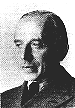Richard von Mises 1883 - 1953

Thus, we are led to the silent assumption, which is not part of the formalized theory, but which is essential for applied probability, that convergence in equation (2) is sufficiently fast that one may judge whether a particular real coin is or is not modelled by the fair coin. The vision and the intuition of the fair coin are fogged over, and the road to axiomatization is beset with pitfalls. So also are the philosophical and psychological bases of probability. There are a good dozen different definitions of a random sequence. The one we have been describing above is that of Richard von Mises, 1919. Let us be a little more precise about this definition. We should like a fair coin to have the H's and T's distributed, in the limit, in a 50%-50% fashion. But we should like more. We should like that if we look at the results of two successive tosses, then each of the four possible outcomes HH, HT, TH, TT occurs, in the limit, with probability 1/4 We should like, shouldn't we, something similar to occur for the eight possible outcomes of three tosses:

HHH, HHT, HTH, HTT, THH, THT, TTH, TTT,

and so on for every conceivable consecutive length of toss. Such a sequence is called ¥ -distributed.
An infinite sequence x1 ,x2, . . . , is called random in the sense of von Mises if every infinite sequence xn1 ,xn2, xn3, . . . extracted from it and determined by a policy or a rule R is ¥ -distributed. Now comes the shocker. It has been established by Joseph Doob that there are no sequences that are random in the sense of von Mises. The requirement is logically self-contradictory.

So one must back down and require less. For practical computing-and "random" sequences are employed quite a bit in programs-one demands quite a bit less. In practise one demands a sequence of integers x1 ,x2, . . . which is easily programmed and inexpensive to produce. The sequence will be periodic but its period should be sufficiently large with respect to the number of random numbers required. Finally, the sequence should be sufficiently crazy and mixed up so as to pass a number of statistical tests for randomness such as frequency tests, runs test, poker hand tests, spectral tests, etc. The program producing such a sequence is called a random number generator, although the successive integers are given by a completely deterministic program and there is nothing, in principle, that is unpredictable about them.

A popular random number generator is given by the formula

xn+1 = kxn(mod m), x0 = 1

This means that each member xn+1 of our random sequence is obtained from its predecessor xn in the sequence by multiplying with a certain number k and then dividing by a certain other number m; xn+1 is the remainder left by this division.

Suppose the computer has a word length of b binary digits or "bits." For k, select a number of the form 8t ± 3, and close to 2b/2. Choose m = 2b The multiplication k . x0 produces a product of 2b bits. The b higher order bits are discarded, leaving the b lower order bits, which is the residue x1. Then repeat the process,

x2 = kx1 (mod m)

and so on. The sequence will not start to repeat itself until the iteration has been carried out 2b-2 times. If the computing machine has a word length of 35 bits, this gives a "random" sequence of roughly 8.5 x 109 numbers.
Further Readings. See Bibliography
H.P. Edmundson; R. von Mises

#### 4. The Aesthetic Component (p168)

"The mathematical sciences particularly exhibit order, symmetry, and limitation; and these are the greatest forms of the beautiful."
Aristotle, Metaphysics, M 3, 1078 b.

The aesthetic appeal of mathematics, both in passive contemplation and in actual research pursuit, has been attested by many authors. Classic and medieval authors, such as Kepler, rhapsodized over the "Divine or Golden Proportion." Poincaré asserted that the aesthetic rather than the logical is the dominant element in mathematical creativity. G. H. Hardy wrote that "The mathematician's patterns, like the painter's or the poet's, must be beautiful. . . ." The great theoretical physicist P. A. M. Dirac wrote that it is more important to have beauty in one's equations than to have them fit the experiment.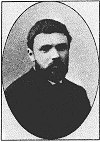Henri Poincaré 1854 - 1912

Blindness to the aesthetic element in mathematics is widespread and can account for a feeling that mathematics is dry as dust, as exciting as a telephone book, as remote as the laws of infangthief of fifteenth century Scotland. Contrariwise, appreciation of this element makes the subject live in a wonderful manner and burn as no other creation of the human mind seems to do.

Beauty in art and in music has been an object of discussion at least since the time of Plato and has been analyzed in terms of such vague concepts as order, proportion, balance, harmony, unity, and clarity. In recent generations, attempts have been made to assign mathematical measures of aesthetic quality to artistic creations. When such measures are built into criteria for construction of musical pieces, say with the computer, it is found that these programs can recapture to a small degree the Mozart-like qualities of Mozart. But the notion of the underlying aesthetic quality remains elusive. Aesthetic judgments tend to be personal, they tend to vary with cultures and with the generations, and philosophical discussions of aesthetics have in recent years tended less toward the dogmatic prescription of what is beautiful than toward discussion of how aesthetic judgments operate and function.

Aesthetic judgment exists in mathematics, is of importance, can be cultivated, can be passed from generation to generation, from teacher to student, from author to reader. But there is very little formal description of what it is and how it operates. Textbooks and monographs are devoid of comments on the aesthetic side of their topics, yet the aesthetic resides in the very manner of doing and the selection of what is done. A work of art, say a piece of colonial Rhode Island cabinetmaking, does not have a verbal description of its unique beauty carved into its mouldings. It is part of an aesthetic tradition and this suffices, except for the scholar.

Attempts have been made to analyze mathematical aesthetics into components- alternation of tension and relief, realization of expectations, surprise upon perception of unexpected relationships and unities, sensuous visual pleasure, pleasure at the juxtaposition of the simple and the complex, of freedom and constraint, and, of course, into the elements familiar from the arts, harmony, balance, contrast, etc. Further attempts have been made to locate the source of these feelings at a deeper level, in psychophysiology or in the mystical collective unconscious of Jung. While most practitioners feel strongly about the importance of aesthetics and would augment this list with their own aesthetic categories, they would tend to be skeptical about deeper explanations.

Aesthetic judgements may be transitory and may be located within the traditions of a particular mathematical age and culture. Their validity is similar to that of a school or period of art. It was once maintained that the most beautiful rectangle has its sides in the golden ratio =  f = 1/2(1 + Ö5). Such a statement would not be taken seriously today by a generation brought up on nonclassical art and architecture, despite experiments of Fechner (1876) or of Thorndike (1917) which are said to bear it out.* The aesthetic delight in the golden ratio f appears nowadays to derive from the diverse and unexpected places in which it arises.

 * Surprisingly, the golden ratio seems to have been taken seriously as an aesthetic principle as recently as 1962 in G. E. Duckworth, Structural Patterns and Proportions in Vergil's Aeneid, Univ. of Michigan Press, 1962. Duckworth analyzes the Aeneid in terms of the ratio M/(M + m), where M is the line length of the "major passages" and m is that of the "minor passages." Duckworth claims that this ratio is .618 (= 1/2(Ö5 - 1)) with fair accuracy.

There is, first, the geometry of the regular pentagon. If the side AB of a regular pentagon has unit length, then any line such as AC has length f = 1/2(1 + Ö5) = 2 cos p/5 = 1.61803...
It appears, next, in difference equations. Take two numbers at random, say 1 and 4. Add them to get 5. Add 4 and 5 to get 9. Add 5 and 9 to get 14. Keep this process up indefinitely. Then, the ratio of successive numbers approachs f as a limit. Witness:1 + 4 = 5 4 + 5 = 9 5 + 9 = 14 9 + 14 = 23 14 + 23 = 37 23 + 37 = 60 37 + 60 = 97 60+ 97= 157 5/4 = 1.250 9/5 =1.800 14/9 =1.555 23/14 =1.643 37/23 =1.608 60/37 =1.622 97/60 =1.617 157/97= 1.618

Finally, continued fractions yield the following beautiful formula: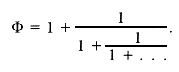What on earth, asks the novice, do these diverse situations have to do with one another that they all lead to f? And amazement gives way to delight and delight gives way to feelings that the universe is united in a wondrous way.

But feelings of a different sort crop up with study and experience. If one works intensively in the theory of difference equations, the unexpected ceases to be unexpected and changes into solid working intuition, and the corresponding aesthetic delight is possibly diminished and certainly transformed. It might even be claimed that situations of "surprise" pose an uncomfortable mystery, which we try to remove by creating a general theory that contains all the particular systems. Thus, all three examples just given for f  can be embraced within a general theory of the eigenvalues of certain matrices. In this way, the attempt to explain (and hence to kill) the surprise is converted into pressure for new research and understanding.
Further Readings. See Bibliography
H. E. Huntley; L. Pacioli

#### 4.Pattern, Order, and Chaos (p172)

A sense of strong personal aesthetic delight derives from the phenomenon that can be termed order out of chaos. To some extent the whole object of mathematics is to create order where previously chaos seemed to reign, to extract structure and invariance from the midst of disarray and turmoil.*
In early usage, the word chaos referred to the dark, formless, gaping void out of which, according to Genesis 1:2, the universe was fashioned. This is what John Milton meant when he used the word in Paradise Lost in paraphrase of the Old Testament:

In the beginning how the heavens and earth
Rose out of Chaos.

In the years since Milton, the word chaos has altered its meaning; it has become humanized. Chaos has come to mean a confused state of affairs, disorder, mixed-upness. When things are chaotic, they are helter-skelter, random, irregular. The opposite of chaos is order, arrangement, pattern, regularity, predictability, understanding. Chaos, in the opinion of the skeptic, is the normal state of affairs in life; in the opinion of the thermodynamicist, it is the state to which things tend if left to themselves.
In view of the ubiquity of chaos, it would be very difficult indeed to give a blanket description to tell whether order or arrangement is or is not present in any given situation; yet it is this presence which makes life comprehensible.

* Otto Neugebauer told the writer the following legend about Einstein. It seems that when Einstein was a young boy he was a late talker and naturally his parents were worried. Finally, one day at supper, he broke into speech with the words "Die Suppe ist zo heiss." (The soup is too hot.) His parents were greatly relieved, but asked him why he hadn't spoken up to that time. The answer came back:"Bisher war Alles in Ordnung." (Until now everything was in order.)

When it is there, we feel it "in our bones," and this is probably a response to the necessities of survival. To create order-particularly intellectual order-is one of the major human talents, and it has been suggested that mathematics is the science of total intellectual order.
Graphic or visual order, pattern, or symmetry has been defined and analyzed in terms of the invariants of transformation groups. Thus, a plane figure possesses axial symmetry around the line y = 0 if it is unchanged (invariant) by the transformation.

{x' = -x .
{y' = y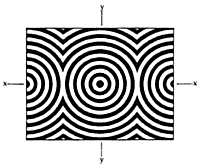A mathematician, like a painter or a poet," wrote G. H. Hardy, "is a master of pattern."
But what can one say about pattern as it occurs within mathematical discourse itself? Are mathematical theories of pattern self referring?

When scientists propose laws of wide generality they set forth rules of law in place of primeval chaos. When the artist draws his line, or the composer writes his measure, he separates, out of the infinitude of possible shapes and sounds, one which he sets before us as ordered, patterned, and meaningful. Consider four possibilities: (1) order out of order; (2) chaos out of order; (3) chaos out of chaos; (4) order out of chaos. The first, order out of order, is a reasonable thing. It is like the college band marching in rank and file and then making neat patterns on the football field during halftime. It is pretty, and the passage from one type of order to the other is interesting, but not necessarily exciting. The second possibility, chaos out of order, is, alas, too common. Some sort of intelligence ends up in a mess. This is the bull in the china shop, an exciting and painful happening. The third possibility, chaos out of chaos, is the bull stomping in the town dump. Despite his intelligence, nothing fundamentally happens, no damage is done, and we hardly notice him at all. The fourth, order out of chaos, is our natural striving, and when we achieve it we treasure it. These four types of transformation will be illustrated with some mathematical patterns or theorems.

Order Out of Order
(a)13 = 12
13 + 23 = (1 + 2)2
13 + 23 + 33 = (1 + 2 + 3)2
13 + 23 + 33 + 43 = (1 + 2 + 3 + 4)2

(b) 4/9 = .4444....
5/37 = .135135135....

(c)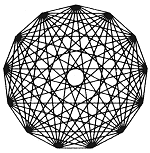(d) The equation of the tangent through (x1,y1) on the conic Ax2 + 2Bxy + Cy2 + 2Dx + 2Ey + F = 0 is Axx1 + B(xy1 + x1y) + Cyy1 + D(x + x1) + E(y + y1) + F = 0.

Chaos Out of Order
(a) 12 x 21 = 252
123 x 321 = 39483
1234 x 4321 = 5332114
12345 x 54321 = 670592745
123456 x 654321 = 80779853376
1234567 x 7654321 = 9449772114007
12345678 x 87654321 = 1082152022374638
123456789 x 987654321 = 121932631112635269

(b) Ö2= 1.4142 13562 37309 50488...

(c) p = 3.1415 92653 58979 32384...

Chaos Out of Chaos
(a) 53278 x 2147 = 114387866

(b)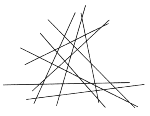(c) (1 + 3x4 - 4x5)(2 - x + 2x2) = 2 - x + 2x2 + 6x4 - 11x5 + 10x6 8x7.

Order Out of Chaos
(a) Pappus' theorem. On two arbitrary lines l1 and l2 select six points P1..... , Q3 at random, three on a line. Then the intersection of P1Q2 P2Q1; P1Q3  P3Q1; P2Q3 P3Q2 are always collinear.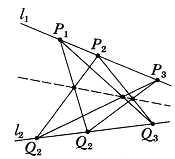(b) The prime number theorem. The sequence of prime numbers 2, 3, 5, 7, 11, 13, 17, 19, 23,. . . occurs in a seemingly chaotic fashion. Yet, if p(x) designates the number of primes that do not exceed x, it is known that lim x®¥ p(x) ¸(x/log x) = 1. That is, the size of p(x) is approximately x/log x when x is large.
Example: if x = 1,000,000,000 then it is known that x/log x = 50,847,478. Now 109/log 109= 48,254,942.43. . .
The function

li(x) = S x0 du/log u

gives even closer results. (See Chapter 5, The Prime Number Theorem).

(c) A polygon whose vertices are random is transformed by replacing it by the polygon formed from the midpoints of its sides. Upon iteration of this transformation, an ellipse-like convex figure almost always emerges. (From: P.J. Davis, Circulant Matrices.)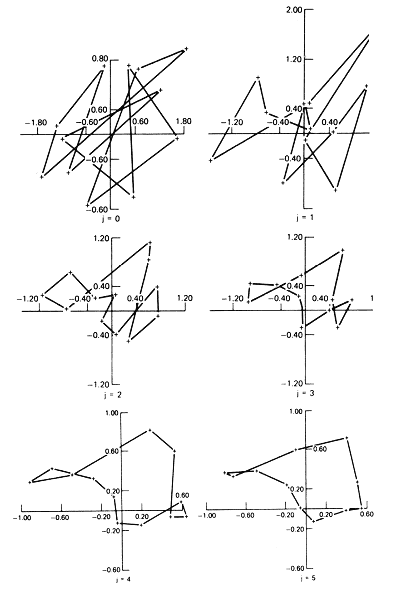(d) Order out of chaos is not always arrived at cheaply. According to an unproved (1979) conjecture of Goldbach (1690-1764), every even number is the sum of two prime numbers. For example, 24 = 5 + 19. This may occur in several different ways: 24 = 7 + 17 = 11 + 13. The accompanying computer listing is of the decomposition of even numbers into the sum of two primes, where the first entry is as small as possible and the last is as large as possible. The chaos is clear. But what is the underlying order? The proof of Goldbach's conjecture, if and when it is forthcoming, may bring order to this chaos.

ILLUSTRATING GOLDBACH'S CONJECTURE

 4 = 2 + 2 6 = 3 + 3 8 = 3 + 5 10 = 3 + 7 12 = 5 + 7 14 = 3 + 11 16 = 3 + 13 18 = 5 + 13 20 = 3 + 17 22 = 3 + 19 24 = 5 + 19 26 = 3 + 23 28 = 5 + 23 30 = 7 + 23 32 = 3 + 29 34 = 3 + 31 36 = 5 + 31 38 = 7 + 31 40 = 3 + 37 42 = 5 + 37 44 = 3 + 41 46 = 3 + 43 48 = 5 + 43 50 = 3 + 47 52 = 5 + 47 54 = 7 + 47 56 = 3 + 53 58 = 5 + 53 60 = 7 + 53 62 = 3 + 59 64 = 3 + 61 66 = 5 + 61 68 = 7 + 61 70 = 3 + 67 72 = 5 + 67 74 = 3 + 71 76 = 3 + 73 78 = 5 + 73 80 = 7 + 73 82 = 3 + 79 84 = 5 + 79 86 = 3 + 83 88 = 5 + 83 90 = 7 + 83 92 = 3 + 89 94 = 5 + 89 96 = 7 + 89 98 = 19 + 79 100 = 3 + 97 102 = 5 + 97 104 = 3 + 101 106 = 3 + 103 108 = 5 + 103 110 = 3 + 107 112 = 3 + 109 114 = 5 + 109 116 = 3 + 113 20882 = 3 + 20879 20884 = 5 + 20879 20886 = 7 + 20879 20888 = 31 + 20857 20890 = 3 + 20887 20892 = 5 + 20887 20894 = 7 + 20887 20896 = 17 + 20879 20898 = 11 + 20887 20900 = 3 + 20897 20902 = 3 + 20899 20904 = 5 + 20899 20906 = 3 + 20903 20908 = 5 + 20903 20910 = 7 + 20903 20912 = 13 + 20899 20914 = 11 + 20903 20916 = 13 + 20903 20918 = 19 + 20899 20920 = 17 + 20903 20922 = 19 + 20903 20924 = 3 + 20921 20926 = 5 + 20921 20928 = 7 + 20921 20930 = 31 + 20899 20932 = 3 + 20929 20934 = 5 + 20929 20936 = 7 + 20929 20938 = 17 + 20921 20940 = 11 + 20929 20942 = 3 + 20939 20944 = 5 + 20939 20946 = 7 + 20939 20948 = 19 + 20929 20950 = 3 + 20947 20952 = 5 + 20947 20954 = 7 + 20947 20956 = 17 + 20939 20958 = 11 + 20947 20960 = 13 + 20947 20962 = 3 + 20959 20964 = 5 + 20959 20966 = 3 + 20963 20968 = 5 + 20963 20970 = 7 + 20963 20972 = 13 + 20959 20974 =11 + 20963 20976 = 13 + 20963 20978 = 19 + 20959 20980 = 17 + 20963 20982 = 19 + 20963 20984 = 3 + 20981 20986 = 3 + 20983 20988 = 5 + 20983 20990 = 7 + 20983 20992 = 11 + 20981 20994 = 11 + 20983 20996 = 13 + 20983 20998 = 17 + 20981 21000 = 17 + 20983